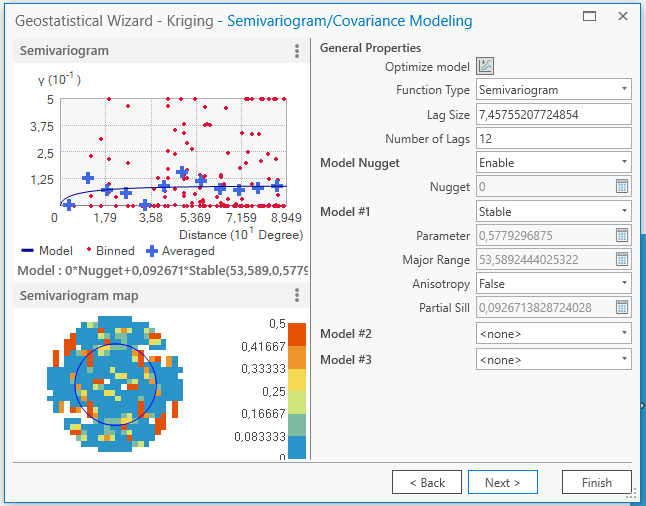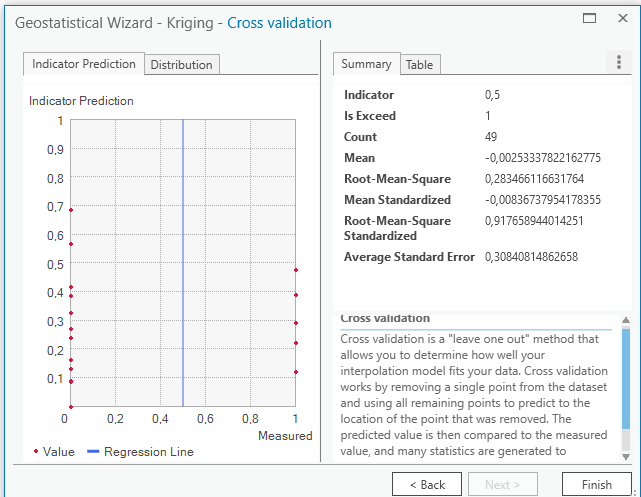# Indicator Kriging in ArcGis Pro (2.6)

72
5
09-18-2020 07:21 AM

Dear all,

I have a set of boring data that I want to use to interpolate to find the hydraulic conductivity of the aquifer. Particularly, I want to look at the semi-impermeable layer that separates the 1st from the 2nd aquifer. I divided my 3D dataset in layers, so that I can work in 2D (which should be OK in a sedimentary aquifer). I have transformed my data into a binary set where 0=sand and 1=clay.

It seems to me this should be a relatively simple 2d indicator kriging exercise. I only find an esri course on EBK online, not on indicator kriging. Thus, I am not sure how to play with the parameters in the geostatistical wizard and I don't know how to interpret for example the semivaiogram and the indicator prediction for a binary dataset.

Does anyone have tips for documentation/tutorials on this? Or does anyone have tips for my work?

Thanks a lot,

SuzanneTags (2)
5 Replies
byEsri Regular Contributor

Hi Suzanne,

In Ordinary (not Indicator) Kriging, the semivariogram is interpreted as the average squared difference in the values of two points, given their distance apart.  Indicator Kriging, however, is slightly different.  The semivariogram in this case is the average squared difference in the indicator values (0 or 1) of two points, given how far apart they are. When the semivariogram value is smaller, this means that the two points more likely to have the same indicator value.

The output is a surface reflecting the probability that a location has an indicator value of 1 (in your case, the probability that the location is clay).

Your second screenshot shows the cross-validation page for indicator kriging.  For each input point, the indicator value is hidden, and the probability that the indicator value of the location is 1 is calculated based on neighbors.  The graph then shows the true indicator value (Measured) plotted against the predicted probability that the point has indicator value equal to 1.  Ideally, points with 0 for the measured value should have low predicted probabilities, and points with 1 for the measured value should have high probabilities.

Unfortunately, that's not quite what I'm seeing in your particular graph.  Points whose true value was 0 (ie, sand points) still have reasonably high predicted probabilities of being clay.  For a few of the points, the indicator kriging model actually thought they were more likely to be clay than sand (these are the red points on the left and above y=0.5).  For the points that actually were clay, the indicator kriging model predicted that they were more likely to be sand than clay (notice that the points on the right all fall below y=0.5).

This can all be a bit confusing, but Indicator Kriging isn't really fundamentally different than the other types of kriging; it just transforms the data into indicator variables first and then proceeds identically.

-Eric

byEsri Regular Contributor

Also, please let me know if you determined whether a point is sand/clay by using a threshold for a continuous variable.  For example, using a density cutoff to classify between sand and clay.

If that's how you created your binary variable, there are a few other methodologies that will likely give more reliable results.

Dear Eric,

thanks a lot for your explanation. What you explain about indicator kriging makes sense and I see that in this case, the indicator kriging did not supply me with a very trustable probability map!

The data that I have, have mainly come from visual analysis of soil samples. So basically, if clay was seen/felt, I recorded clay, if sand was seen I recorded sand. I also have some data from cone penetration tests (measuring resistance, with also gives me a soil classification more than an actual hydraulic conductivity K value). I also used publicly available data on the local aquifers' conductivities. In the set of K values I created from this data, clay has a value of 0.005 m/d and sand ranged from (a little bit of) fine sand (3 m/d), through to sand (15 m/s), all the way to course (50 m/d) and very course (80m/d) sand with pebbles.

I am not sure how I could improve my data in order to get better kriging results.. but curious to hear what you think!

Suzanne

byEsri Regular Contributor

It may not work with your data, but the idea I had was to Empirical Bayesian Kriging with a Probability map output.  If you had a continuous variable with a threshold indicating the cutoff between sand and clay, you could just interpolate the continuous variable with EBK and calculate the probability that each new location exceeds that threshold using the predicted value and the standard error.  The Probability output type does this calculation automatically.

The biggest problem with Indicator Kriging is that when you reclassify from a continuous variable to a binary variable, you lose lots of information.  This is why Indicator Kriging usually doesn't perform well.  But if you only have indicator variables to start out and didn't create them with a reclassification, indicator kriging is about the best you can do.

And just to add to the complexity, there's another type of kriging called Probability Kriging.  It takes a continuous variable and a threshold.  It then reclassifies the values to 0 and 1 and performs indicator kriging.  However, it also uses the raw values of the continuous variable as a cokriging variable.  This essentially tries to bring back in some of the information that was lost during the reclassification.  In my experience, just using EBK with the Probability output type almost always yields best results (again, if you have a continuous variable), but there are a bunch of options available.

Hi Eric, thanks, I'll have a look at where I get to with EBK and let you know!